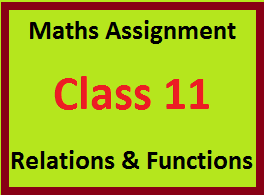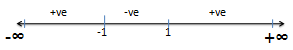### Resource Centre Mathematics

Resource Centre Mathematics Mathematics worksheet, mathematics basic points and formulas, mathematics lesson plan, mathematics multiple choice questions Workplace Dashboard CBSE Syllabus For Session 2023-24 For  :   Classes IX & X    |   Classes XI & XII Watch Videos on Maths Solutions CLASS IX MATHEMATICS FORMULAS &  BASIC CONCEPTS

## MATHEMATICS ASSIGNMENTChapter 2 Class -11Relations and Functions

Extra questions of chapter 2 class 11  with answer and  hints to the difficult questions. Important and useful math. assignment for the students of class 11## ASSIGNMENT ON RELATIONS & FUNCTIONS

LEVEL-1

Question 1

i)  Find x and y if  (x + 3, 5) = (6, 2x + y)

Ans (x = 3, y = - 1)

ii) If ordered pair (x, -1) and (5, y) belongs to the set {(a, b): b = 2a - 3}, find the value of x and y

iii) Find the value of a and b If

[Ans a = 2, b = 1]

(iv) If a  {-1, 2, 3, 4, 5}, b  {0, 3, 6}, write the set of all ordered pairs (a, b) such that
a + b = 5
Solution
-1 + 6 = 5
(-1, 6)  (a, b)
2 + 3 = 5
(2, 3)  (a, b)
5 + 0 = 5
(5, 0)  (a, b)
R = {(-1, 6), (2, 3), (5, 0)}

Question 2

i)  If A = {1, 3, 5, 6} and B = {2, 4}, find A x B and B x A

ii) If A = {1, 2. 3}, B = {3, 4} and C ={1, 3, 5}, find

(a) A x (B ∩ C)

(b)  (A x B) ∩ (A x C)

iii) If A = {1, 3, 5} , B = {x, y} then represent A x B and B x A in arrow diagrams

Question 3

Find the inverse relation R-1 in each of the following

(i) R = {(1,2), (1,3), (2, 3), (3, 2), (5, 6)

Ans {(2,1), (3,1), (3,2), (2,3), (5,6)}

(ii) R = {(x, y) : x, y  N, x + 2y = 8

Ans {(3, 2), (2, 4), (1,6)

Solution
If  x = 2, y = 3
2 + 2 x 3 = 8  (2, 3)  R
If  x = 4, y = 2
4 + 2 x 2 = 8  (4, 2)  R
If  x = 6, y = 1
6 + 2 x 1 = 8  (6, 1)  R
R = {(2, 3), (4, 2), (6, 1)}
{(3, 2), (2, 4), (1, 6)}

Question 4

Find the domain and range of the relation R defined by
R = {(x,  x) : x is a prime number less than 10}

Ans[Domain R = {2, 3, 5, 7},
Range R = {8, 27, 125, 343}]

Question 5

(i) n(A) = 3, n(B) = 4, then find  n(A x A x B)

Ans: 36

(ii) If A = {1, 2, 4}, B = {2, 4, 5) and C = {2, 5}, write (A - C) x (B - C)

Ans : {(1, 4), (4, 4)}

(iii)  If A = {1, 2} and  B = {3, 4}. Find A x B and total number of subsets of A x B. Also find the total number of relations from A to B

[Ans:    22 ✕ 2  = 2=16 ]

(iv)   If A = {1, 2, 3, 5}, B = {4, 6, 9} and R be a relation from A to B  defined by

R = {(x, y): |x - y|  is odd}. Write R in roster form.

Ans:  R = {(1, 4), (1, 6),  (2, 9), (3, 4), (3, 6), (5, 4), (5, 6)

Question 6

Express the following functions as set of ordered pair and determine their ranges

(i)   f : A R, f(x) = x+ 1,  where A = {-1, 0, 2, 4}

(ii)  g : A N, g(x) = 2x, where A = { x : x N, x ≤ 7}

[Ans(i): Range = {1, 2, 5, 17} ]

[Ans(ii) : Range = {2, 4, 6, 8, 10,12,14}

Question 7

Let f : R - {2}  R be defined byand g : R  R be defined by g(x) = x + 2. Find whether f = g or not.

Solution:

Also g(x) = x + 2   f(x) = g(x)

But  Domain of f(x) = R - {2}  and the domain of g(x) = R  and two functions

are equal only if their domains are equal.

Hence f(x) ≠ g(x)

Question 8

Find the domain for which the functions f(x) = 2x2 - 1 and g(x) = 1 - 3x are equal

Solution:

Here f(x) = g(x)

2x2  - 1 = 1 - 3x    2x2 + 3x – 2 = 0

x = -2, 1/2

f(x) and g(x) are equal on the set  {-2, 1 / 2 }

Question 9

Let f : RR be a function given by f(x) = x+ 1. Find    -1{10, 37}

Solution

If f(x) = y then  x = f -1(y)

-1(10) = x  f(x) = 10  x+ 1 = 10

x= 9   x =  3

f -1(37) = x  f(x) = 37  x+ 1 = 37

x= 36   x =  6

f -1{10, 37} = {-3, 3, - 6, 6}

Question 10

Let A = {-2, -1, 0, 1, 2} and f : A  Z be a function defined by  f(x) = x- 2x - 3. Find

(i) Range of f i.e. f(A)          (ii) Pre - image of 6, - 3 and 5

Solution

(i)  f(A) = {f(-2), f(-1), f(0), f(1), f(2) } = {5, 0, - 3, - 4, - 3} = {- 4,- 3, 0, 5}

(ii) Let Pre - image of 6 = x   -1(6) = x  f(x) = 6

x2 - 2x - 3 = 6     x- 2x - 9= 0

There is no real value of x which satisfies this equation.

So Pre - image of  6 = Φ

Let Pre - image of - 3 = x   -1(-3) = x  f(x) = - 3

x2 - 2x - 3 = - 3     x2 - 2x = 0   x = {0, 2}

Let Pre - image of 5 = x   -1(5) = x  f(x) = 5

x2 -2x - 3 = 5    x2 - 2x - 8 = 0   x = {-2, 4}

## ASSIGNMENT ON RELATIONS & FUNCTIONS

Level - 2

Question 11

If f, g, h are three functions defined from R to R as follows

(i) f(x) = x2      (ii)  f(x) = sinx    (iii) f(x) = x2 + 1

Find the range of each function

Solution

(i)   For all values of x,  f(x) takes only   +ve value. Also if x = 0, then f(x) = 0 . So Range  of  f(x)  =  [0, ∞)

(ii) Since  -1 ≤ sinx ≤ 1 for all values of x. So Range of f(x) = [-1,1]

(iii) Since  x2 is ≥ 0  x+ 1 ≥ 1   Range of f(x) = [1, ∞)

Question 12  If  then find f(f(x))

Solution:

Question 13

Find the domain of the function f(x) defined by

Solution

f(x) is defined for all x satisfying

4 - x ≥ 0 and x- 1 > 0

x ≤ 4 and (x - 1)(x + 1) > 0
For (x - 1)(x + 1) = 0, the critical points are x = 1 and x = -1

(x - 1)(x + 1) > 0x(- ∞, -1) U (1, ∞),   But   x ≤ 4

Therefore : Df  = (- ∞, -1) U (1, 4]

Question 14

Find the domain and range of the functions

Solution

By using quadratic formula we get

Now x is defined (real) if 1 - 4y2 ≥ 0 and y ≠ 0

4y2 - 1 ≤ 0     (2y+1)(2y - 1) ≤ 0

Critical points are  y = -1/2 and  1/2(2y + 1)(2y - 1) ≤ 0   and  y ≠ 0  y  [-1/2, 1/2] - {0}

Rf = [-1/2, 1/2] - {0}

(ii)  Find the domain and range of the functions

(iii)  Find the domain and range of the functions

Solution (iii)

f(x) is defined for all real numbers except at x = 3

Therefore Df = R - 3

y = f(x) =

But x = 3  Df    y = 3 + 3 = 6  Rf    Rf = R - {6}

Question 15

Find the domain and range of the following functions

i) f(x) = |x-1|        Ans : [D = R]  R = [0, ∞)

ii) x2 + y2 = 25

iii)

Ans:

iv)

Ans: Domain = R-{2}, Range = {-1}

v)

Ans: Domain = [-4, 4], Range = [0, 4]

vi)

Ans: Domain = (-3, 3) Range = [1, ∞)

Solution (vi)

f(x) is defined if

⇒ 9y- x2y= 9

⇒ x2y2 = 9y2 - 9

x is defined if  9y- 9 ≥ 0 and  y ≠ 0
x is defined if  y- 1 ≥ 0 and  y ≠ 0

x is defined if  (y + 1)(y - 1) ≥ 0 and  y ≠ 0

x is defined if  y ≤  -1,   y ≥ 1 and  y ≠ 0

⇒  y ∈ (-∞, -1] ⋃ [1, ∞)
⇒ Rf  = (-∞, -1] ⋃ [1, ∞)

(vi) Find the domain of the function

Ans: Df  = (-∞, -3] ⋃ (-1, 3]

Question 16

(i) f(x) = 3x- 5x+ 9, find  f(x - 1)

Ans[3x- 12x+ 13x2  - 2x + 7]

(ii) Write the domain of f(x) = x+ 1 and draw its graph. Also find the value of x for which  f(x) = f(x + 1)

(iii) If f(x) = x- 3x + 4, then find the value of  x such that f(x) = f(2x + 1)

[Ans x = -1, 2/3]

(iv) F(x) = 4x - x2, x   R, then find  f(a + 1) – f(a - 1)

Question 17

Draw the graph of the following write its range

Question 18

If     then evaluate f(-2) + f(2)

Question 19

Find the domain of the following function

Ans : Df  = R - {1, 2}

Question 20:  Solve :

Ans:

Solution: Let |x| = y

Critical points are  y = 5, y = 3

y = |x| = 5  and  y = |x| = 3  ⇒  x = 土 5,  x = 土 3

Question 21:
Let f = {(1,1), (2,3), (0,-1), (-1, -3)} be a linear function from Z ⟶ Z. Find the function f as a linear function of x.
Solution: As given f is a linear function, let f(x) ax + b. Also (1,1) and (2,3) ∊ f
f(1) =1 and f(2) = 3
⇒ a + b = 1 and 2a + b = 3
On solving these equations  we get a = 2, b = -1
⇒ f(x) = 2x - 1

Question 22
Find the domain and range of
Ans: Domain of f  = R-{√2, -√2}
Range of f = [3/2, ∞) ∪ (-∞,0]

Question 23
Draw the graph of the function f defined by f(x) = x + |x + 1|. Hence find the range from the graph.